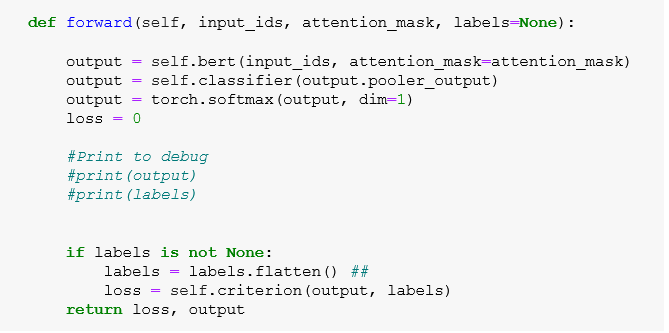# ValueError while running multi-class predictions

I am doing multi-class predicition with softmax and CrossEntropyLoss. My output is [0.4559, 0.2230, 0.3211] and label is . I am very new to pytorch and have no idea how to correct this error I am getting: ValueError: If `preds` and `target` are of shape (N, …) and `preds` are floats, `target` should be binary. Any suggestions would be really appreciated.

The task that you are working on seems a little bit ambiguous for me. So I would be more helpful if you give more information, like, what is your batch size? what is your exact dimensions of target and prediction tensor shapes?

Assuming tensors as you say: `preds: [0.45, 0.22, 0.32], target: 1`, the corresponding loss calculation should be

``````import torch

criterion = torch.nn.CrossEntropyLoss()
criterion(torch.tensor([0.45, 0.22, 0.32]).unsqueeze(1).unsqueeze(0), torch.tensor().unsqueeze(0).long())
#tensor(1.2131)
``````

where .unsqueeze(0) for batch size of 1 and .unsqueeze(1) is for sequence length.

``````print(torch.tensor([0.45, 0.22, 0.32]).unsqueeze(1).unsqueeze(0).shape)
# torch.Size([1, 3, 1])

print(torch.tensor().unsqueeze(0).long().shape)
# torch.Size([1, 1])
``````

I hope this would be helpful.

Hi Safak,

Many thanks for your response. My batch size is 12, dim=1. An example tensor shape for my output is torch.Size([10, 3]) and for my labels it is torch.Size([10, 1]). I applied labels.flatten() - it did not show the error and calculated the loss. See below the code:Predictions look ok to me but need to convert it into the label (get the max index). Do you know the best way of doing that? Thanks

If your batch size is 12, sequence length is 10 and number of your classes is 3; your output before passing it loss fn should have dimension `[batch_size, n_classes, seq_len]` and your target should have dimension `[batch_size, seq_len]`. An example: `[12, 3, 10]` and `[12, 10]`. It is okey to pass this to `nn.CrossEntropyLoss` without any flattening.

If you have batch size at dimension 1; y_pred: `[10,12,3]` and target: `[10,12,1]`, you can pass it like `criterion(y_pred.permute(1,2,0), target.permute(1,2,0).squeeze(1))`

``````print(y_pred.permute(1,2,0).shape)
# torch.Size([12, 3, 10])

print(target.permute(1,2,0).squeeze(1))
# torch.Size([12, 10])
``````

After organizing your shapes, you can get your highest probability classes with

``````print(y_pred.permute(1,2,0).shape)
# torch.Size([12, 3, 10])

argmax_preds = torch.argmax(y_pred.permute(1,2,0), dim=1)
``````

``````accuracy = (argmax_preds == target).cpu().numpy().mean() * 100